# Competing With Gaussian Linear Experts

This paper describes an approach to prove guarantees on the loss of online Ridge Regression. The results of the paper are approached from the probabilistic perspective in [An Identity For Kernel Ridge Regression | Zhdanov and Kalnishkan, 2010]].

Theorem. The Ridge Regression algorithm for the learner with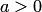satisfies, at any step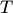,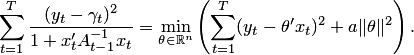The following corollary on the upper bound of clipped Ridge Regression follows from the theorem.

Corollary. Assume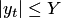for all, clip the predictions of Ridge Regression to, and denote them by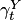. Then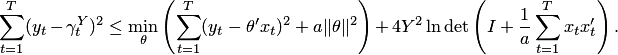The approach to prove the main theorem is very elegant. The Ridge Regression predictions are considered as the means of the Bayesian Ridge Regression predictions, and the loss bound is easily derived from the loss bound on the Bayesian Ridge Regression. The loss bound on the logarithmic loss of the Bayesian method in the form of equality is proved using the Bayesian algorithm, a special case of the Aggregating Algorithm. The Bayesian algorithm competes with Gaussian Linear Experts. The following bound is proven.

Theorem. For any sequence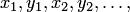the cumulative logarithmic loss of the Bayesian Ridge Regression algorithm at any stepcan be expressed as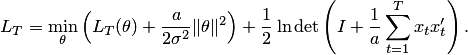If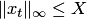for any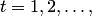then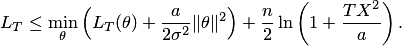The guarantees for the kernelized version are also proven.

• Fedor Zhdanov and Vladimir Vovk. Competing with Gaussian linear experts. Technical report, arXiv:0910.4683 [cs.LG], arXiv.org e-Print archive, 2009.
• Fedor Zhdanov and Yuri Kalnishkan. An identity for kernel ridge regression. In Proceedings of the 21st International Conference on Algorithmic Learning Theory, 2010.